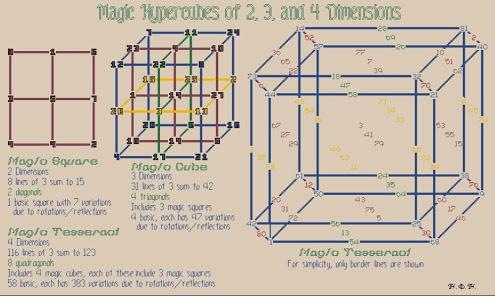# What Do You Know About Magic Hypercubes?

10 Questions | Total Attempts: 102SettingsIn the field of mathematics, magic hypercubes are k-dimensional conceptions of magic squares, magic cubes, and magic tesseracts that increase their dimensions. Now, let's see what you know about magic hypercubes by taking this short, intelligent quiz.

• 1.
Which of the following is denoted as the magic constant in magic hypercubes?
• A.

Mk(n)+2

• B.

Mk(n)+1

• C.

Mk(n)

• D.

Mk(n)-1

• 2.
Out of all the theorems used in hypercube, who proved the one below? 'A p-dimensional magic hypercube of order n exists if and only if p > 1 and n is different from 2 or p = 1.'
• A.

Marian Trenkler

• B.

John Hendricks

• C.

James Stoner

• D.

Kathleen Ollerenshaw

• 3.
What is the directions within the hypercube called?
• A.

Pathfinders

• B.

Digit changing

• C.

Reflection

• D.

Aspect

• 4.
What is the formula of Aspectical variants?
• A.

NH~R perm(0..n+2); R = ∑n-1 ((reflect(k)) ? 2k : 0) ; perm(0..n-1) a permutation of 0..n-1

• B.

NH~R perm(0..n+1); R = ∑n-1 ((reflect(k)) ? 2k : 0) ; perm(0..n-1) a permutation of 0..n-1

• C.

NH~R perm(0..n-1); R = ∑n-1 ((reflect(k)) ? 2k : 0) ; perm(0..n-1) a permutation of 0..n-1

• D.

NH~R perm(0..n-2); R = ∑n-1 ((reflect(k)) ? 2k : 0) ; perm(0..n-1) a permutation of 0..n-1

• 5.
What is described as the change of [i] into [perm(i)] alongside the given "axial"-direction?
• A.

Monogonal permutation

• B.

Digitchanging

• C.

Component permutation

• D.

KnightJump construction

• 6.
Which formula denotes the Latin prescription construction—modular equations?
• A.

LP = ( ∑n-2 LP x + LP ) % m

• B.

LP = ( ∑n+2 LP x + LP ) % m

• C.

LP = ( ∑n+1 LP x + LP ) % m

• D.

LP = ( ∑n-1 LP x + LP ) % m

• 7.
Which procedure makes use of the isomorphism of every hypercube that changes the hypercube?
• A.

Dynamic numbering

• B.

Monogonal permutation

• C.

Digitchanging

• D.

Pathfinders

• 8.
Who proved the theorem that 'a p-dimensional magic hypercube of order n exists if and only if p > 1 and n is different from 2 or p = 1'?
• A.

C. Planck

• B.

Marian Trenkler

• C.

Samuel Walker

• D.

J. R. Hendricks

• 9.
Which procedure is used when calculating the sum for p-multimagic hypercubes?
• A.

Faulhaber's formula and divide it by mn-1

• B.

Faulhaber's formula and divide it by mn-2

• C.

Faulhaber's formula and divide it by mn+1

• D.

Faulhaber's formula and divide it by mn+2

• 10.
Which of the following is a variation of magic hypercubes?
• A.

Faulhaber magic hypercubes

• B.

Nasik magic hypercubes

• C.

Marian magic hypercubes

• D.

Planck magic hypercubes

Related TopicsBack to top